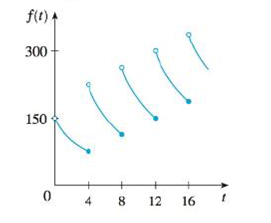Chapter 2.2, Problem 10E### Single Variable Calculus: Early Tr...

8th Edition
James Stewart
ISBN: 9781305270343

#### Solutions

Chapter
Section### Single Variable Calculus: Early Tr...

8th Edition
James Stewart
ISBN: 9781305270343
Textbook Problem

# A patient receives a 150-mg injection of a drug every 4 hours. The graph shows the amount f(t) of the drug in the bloodstream after t hours. Find lim t → 12 − f ( t ) and lim t → 12 + f ( t ) and explain the significance of these one-sided limits.To determine

To find: The value of limt12f(t) and limt12+f(t); explain the meaning of the one-sided limits.

Explanation

From the given graph, it is found that the curve takes the value of 150 from the left side.

That is, limt12f(t)=150 mg.

The left-sided limit represents the amount of drug in patient’s bloodstream before receiving the fourth injection.

Also, it is found that the curve takes the value of 300 from the right side

### Still sussing out bartleby?

Check out a sample textbook solution.

See a sample solution

#### The Solution to Your Study Problems

Bartleby provides explanations to thousands of textbook problems written by our experts, many with advanced degrees!

Get Started